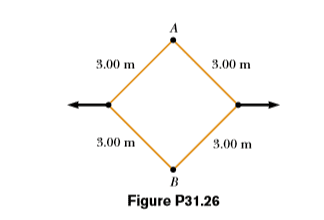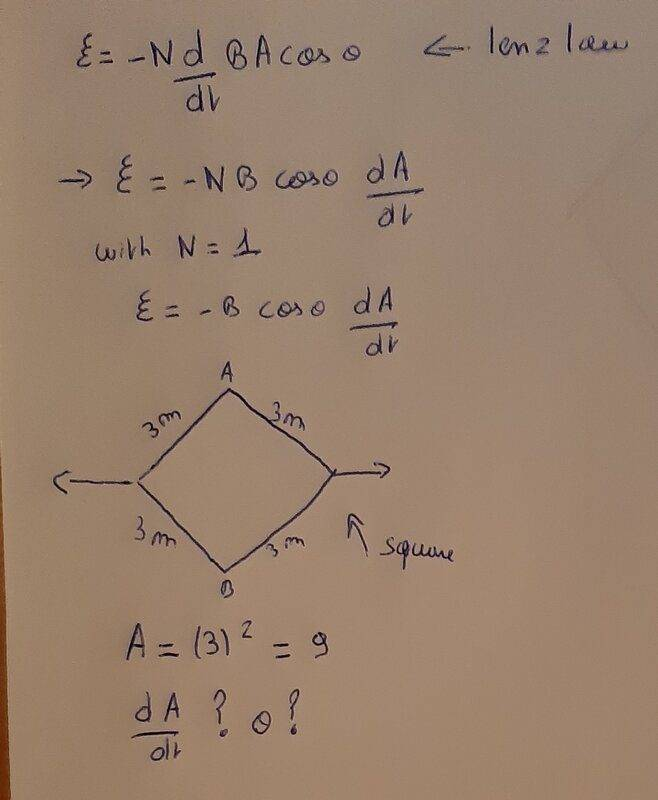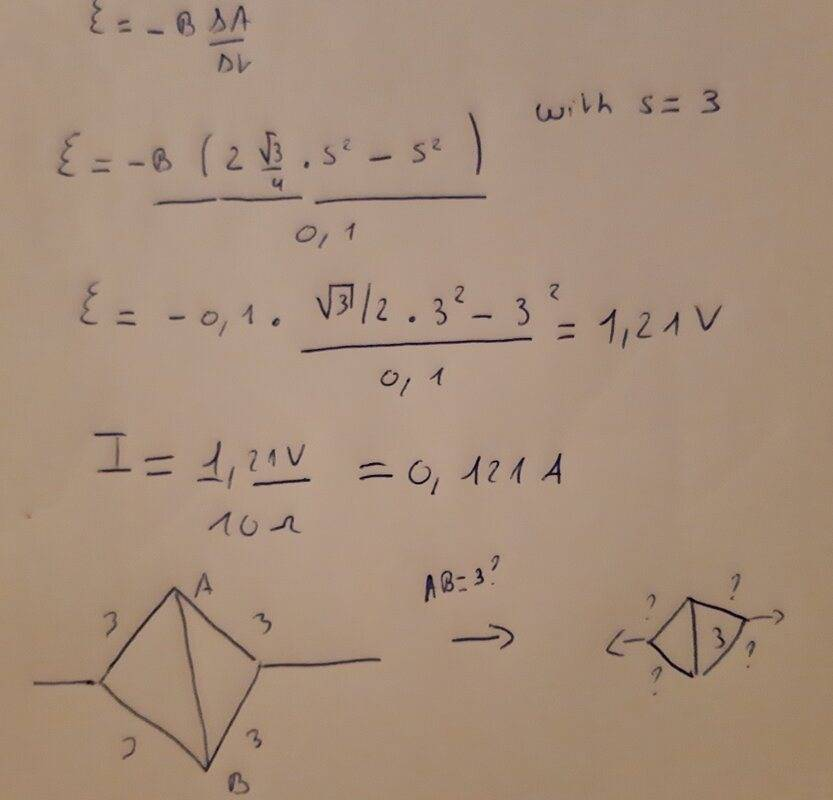# Lenz law how to find dA/dT and O?

• Nicolas01

#### Nicolas01

Homework Statement
The square loop in Figure P31.26 is made of wires with total series resistance 10.0 (. It is placed in a uniform 0.100-T magnetic ﬁeld directed perpendicularly into the plane of the paper. The loop, which is hinged at each corner, is pulled as shown until the separation between points A and B is 3.00 m. If this process takes 0.100 s, what is the average current generated in the loop? What is the direction of the current?
Relevant Equations
Lenz lawHello,
I am new and I have a question about the lenz law. How I am suppose to find DA/dt and o in this problem? A= 9 but D(9)/dt is equal to 0 ? And I don't really know what is the angle o means in this formula. If I found the voltage i can find the current with I= eps/Req .

It won't work if you just differentiate a value, you must be working with functional forms. Since ##\cos{\theta} = \vec{B} \cdot \vec{A} = 1##, your equation for EMF will reduce to $$\mathcal{E} = -B\frac{dA}{dt}$$Since it asks for average current, you can work out the average EMF by changing that derivative to a ratio of finite changes,$$\langle \mathcal{E} \rangle = -B\frac{\Delta A}{\Delta t}$$It is not clear to me what the starting configuration is. Are we to assume that initially the distance between A and B is zero (i.e. the initial area enclosed is zero), and that we pull them apart until they are at a separation of ##3 \text{m}##? If so, you should be able to calculate the change in area during the process (the final state is two equilateral triangles) and with ##\langle \mathcal{E} \rangle## you can find ##\langle I \rangle## with Ohm's law.

•Nicolas01
It won't work if you just differentiate a value, you must be working with functional forms. Since ##\cos{\theta} = \vec{B} \cdot \vec{A} = 1##, your equation for EMF will reduce to $$\mathcal{E} = -B\frac{dA}{dt}$$Since it asks for average current, you can work out the average EMF by changing that derivative to a ratio of finite changes,$$\langle \mathcal{E} \rangle = -B\frac{\Delta A}{\Delta t}$$It is not clear to me what the starting configuration is. Are we to assume that initially the distance between A and B is zero (i.e. the initial area enclosed is zero), and that we pull them apart until they are at a separation of ##3 \text{m}##? If so, you should be able to calculate the change in area during the process (the final state is two equilateral triangles) and with ##\langle \mathcal{E} \rangle## you can find ##\langle I \rangle## with Ohm's law.Thanks i got the right answer but i don't know how you found that final state is two equilateral triangles? Because if AB=3 what the length of the other sides. Thanks again

Thanks i got the right answer but i don't know how you found that final state is two equilateral triangles? Because if AB=3 what the length of the other sides. Thanks again

All of the wires are given to be of length ##3\text{m}##•Nicolas01
All of the wires are given to be of length ##3\text{m}##All of the wires are given to be of length ##3\text{m}##Ok i got it, thanks you for helping ! Have a nice day

•etotheipi